§3

1. 基本概念

[导数的定义及其几何意义]  设函数y=f(x)当自变量在点x有一改变量,函数y相应地有一改变量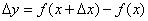,那末当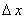趋于零时,若比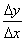的极限存在(一确定的有限值)，则称这个极限为函数f(x)在点x的导数,记作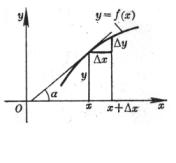图5.1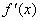=[单边导数]=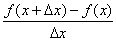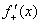=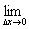=[无穷导数]  若在某一点x=±∞

+∞时,函数f(x)的图形在点x的切线正向与y轴方向一致,=－∞时,方向相反)

[函数的可微性与连续性的关系]  如果函数y=f(x)在点x有导数，那末它在点x一定连续。反之,连续函数不一定有导数,例如

函数y=|x|在点x=0连续,在点x=0,左导数=1,右导数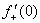=1,而导数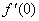不存在(5.2)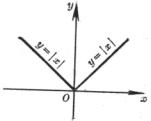`图5.2            图5.3`

函数

y=f(x)=